# Qlik Sense Documents

Qlik Sense documentation and resources.

Announcements
Support Case Portal has moved to Qlik Community! Read the FAQs to start exploring Support resources.

# Calculating trend lines, values and formulas on charts and tables in Qlik Sense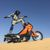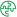Contributor III

## Calculating trend lines, values and formulas on charts and tables in Qlik Sense

I spent a little time working through the formulas required to mimic the trend lines that are available in QlikView and currently not available in Qlik Sense without knowing how to write reasonably complex expressions.

Here are some examples of exponential and 2nd order polynomial trend lines with the relationship expressed as a formula in the subtitle.

Exponential Trend Line2nd order polynomial trend line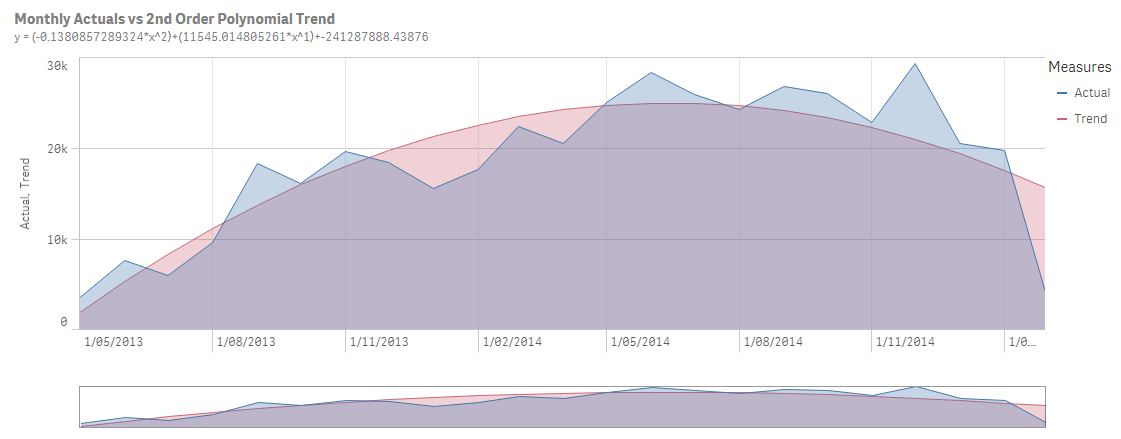To make things as clear as possible I have included the Excel variants of these formulas so you have something to reference as I have done whilst checking these formulas calculate correctly. These excel formulas were sourced from Excel Tips From John Walkenbach: Chart Trendline Formulas

The below table shows the equivalent formulas in Qlik for the excel formulas provided. I have also attached a QVF file with examples of each one so that you have something real to reference as you build these into your own applications. In addition I have included the excel file that I was using for testing to ensure my calculations were correct.

## Qlik Formulas

### Linear Trendline

Equationy = m * x + by = m * x + b
m= SLOPE(y,x)= LINEST_M(y,x)
b= INTERCEPT(y,x)= LINEST_B(y,x)

### Logarithmic Trendline

Equationy = (c * LN(x)) + by = (c * LOG(x)) + b
c= INDEX(LINEST(y,LN(x)),1)= LINEST_M(y,LOG(x))
b= INDEX(LINEST(y,LN(x)),1,2)= LINEST_B(y,LOG(x))

### Power Trendline

Equationy=c*x^by = c * POW( x , b)
c= EXP(INDEX(LINEST(LN(y),LN(x),,),1,2))= EXP(LINEST_B(LOG(y),LOG(x)))
b= INDEX(LINEST(LN(y),LN(x),,),1)= LINEST_M(LOG(y),LOG(x))

### Exponential Trendline

Equationy = c *e ^(b * x)y = c * POW( e , b * x)
c= EXP(INDEX(LINEST(LN(y),x),1,2))= EXP(LINEST_B(LOG(y),x))
b= INDEX(LINEST(LN(y),x),1)= LINEST_M(LOG(y),x)
e= EXP(1)= e()
2nd order Polynomial Trend
Equationy = (c2 * x^2) + (c1 * x ^1) + by = (c2 * POW(x,2)) + (c1 * x) + b
c2= INDEX(LINEST(y,x^{1,2}),1)see example - variable c2
c1= INDEX(LINEST(y,x^{1,2}),1,2)see example - variable c1
b= INDEX(LINEST(y,x^{1,2}),1,3)see example - variable b

### Points to note:

1. You will see in the QVF that I have used monthly aggregated values from more detailed data which is a real life requirement (you would not necessarily want to create an aggregated table just for this purpose). Therefore the Y values are shown aggregated by the dimension. i.e. aggr(Sum([Expenses (USD)]),MonthYear)
2. The 2nd Order Polynomial trend is a little more complex than the others. I have not found a comparable function to excel LINEST(Y,x^{1,2}) so have managed to find some old examples and put together a longhand version. Please see the variables in the example application.
3. The 2nd Order Polynomial dimension has some specific requirements in the current form of the expressions. It MUST be a field with distinct values in the dataset, i.e. MonthYear in my example must have the grain of MonthYear and not be a field in the calendar table. It should also join directly to the fact where actuals reside, not join through another dimension.Contributor II

Thanks, this is really useful.Contributor

Sorry for my English.

Can it be more accurate?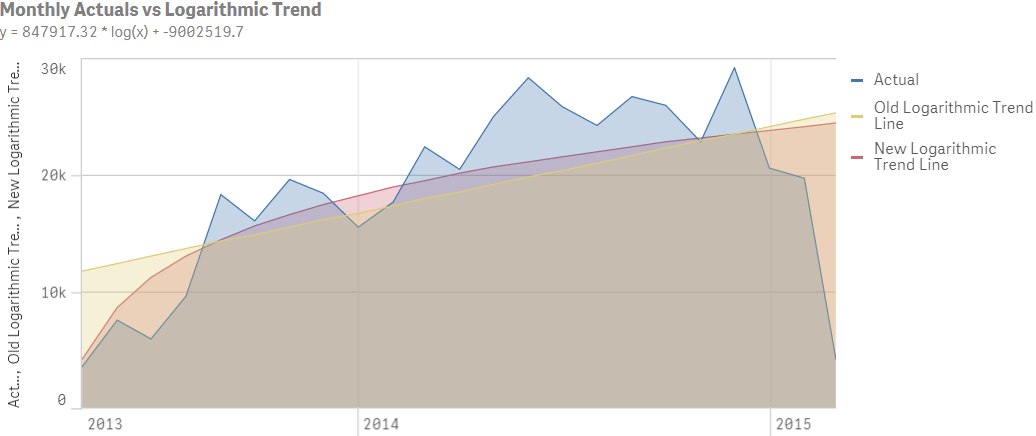linest_m(total aggr(sum(Expenses),MonthYear),(Aggr(Log(Rowno()) * MonthYear, MonthYear)))

* (Aggr(Log(Rowno()) * MonthYear, MonthYear))

+ linest_b(total aggr(sum(Expenses),MonthYear),(Aggr(Log(Rowno()) * MonthYear, MonthYear)))Contributor III

Hi Andy,

Great to share different options so we can find the best fit for our needs but I'd be cautious with your use of the term 'accurate'. To me the yellow line is more representative of the trend than the red one but that is subjective...

If you solve the 3rd an 4th order polynomial lines please let me know as this was a step too far for me 😉

Cheers

RichardPartner

Hi Richard,

Thanks for your solution on the 2nd order polynomial. In your comments you wrote that  there is no equivalent INDEX(y,x^{1\2},1). I'm not sure if you already know that {1\2} is an extra x -column. For example:

LINEST_M equals the LINEST function in Excel.

for the following table:

 Y value X value 0,6931 1 0,7581 2 0,7553 3

the LINEST(Y:Y, X:X) = 0.0311. Same goes for LINEST_M in Qlik.

Adding the ^{1\2] leads to the following table in Excel (first x^1 and second x^2)

 Y value X value X-2 value 0,6931 1 1 0,7581 2 4 0,7553 3 9

With LINEST(Y:Y ; X:X-2) you'll get -0.0399 which is your C2 value.

So the LINEST_M in Qlik comes close to the LINEST function in Excel only I don't exactly know how to get two X-columns into the LINEST_M function. Maybe you are able to figure it out.

If you already knew about the two X-columns than you can ignore my post of course.

Anyway thank you very much for the calculations.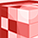Creator III

Hi all,

in a scatterplot where my measures are y=sum(sales) and x=sum(profit) and i have one dimension

my linear regression line would be

linest_m(total aggr(sum(sales),dimension)), aggr(sum(profit),dimension)) * sum(profit) + linest_b(total aggr(sum(sales),dimension)), aggr(sum(profit),dimension))  ?

Version history
Revision #:
1 of 1
Last update:
‎2017-10-03 07:08 AM
Updated by: